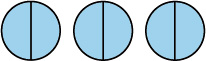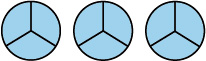Succeed with maths – Part 1

Start this free course now. Just create an account and sign in. Enrol and complete the course for a free statement of participation or digital badge if available.

Free course

# 3 Introduction to dividing fractions

Dividing fractions is a little more difficult, so you’ll start by considering simple examples. You’ll then attempt to translate that to a more complex question by applying a similar strategy.

Suppose that you have three pizzas, and you divide each of them in half (see Figure 5).Figure _unit5.3.1 Figure 5 Three circles, each divided into half

How many halves do you have? You can see that there are six halves in three pizzas. Thus this shows how many halves there are in three. This is the same as dividing 3 by a . When you calculate , you can think of this as ‘How many halves are there in three?’ Here are few similar examples to work out how to divide by fractions. There are three pizzas again, but this time the question is 'How many thirds can they be cut up into?' (Figure 6). This is the same as asking what is .Figure _unit5.3.2 Figure 6 Three circles, divided into thirds

From this, you can see that there are 9 thirds in 3. So .

Now divide these three pizzas into quarters, which gives the answer to . Each pizza will be cut into four parts to give quarters and there are three pizzas, so the total number of slices will be .

You also know that . Looking at these three calculations, can you see a way of arriving at the answers without drawing out a picture to help? Look at the whole numbers and the denominators.

You may have noticed that if you multiply these together, then you arrive at the answer.

So it seems that , and .

So put into words, you can say that to divide by a fraction, you have to swap the numerator and the denominator, and then multiply.

Or, in mathematical language (so that you can communicate this clearly and concisely to others): when the numerator and denominator change places, the result is called the reciprocal of the original fraction. Thus, dividing by a fraction is the same as multiplying by its reciprocal.

In the next section you’ll look at this in practice.